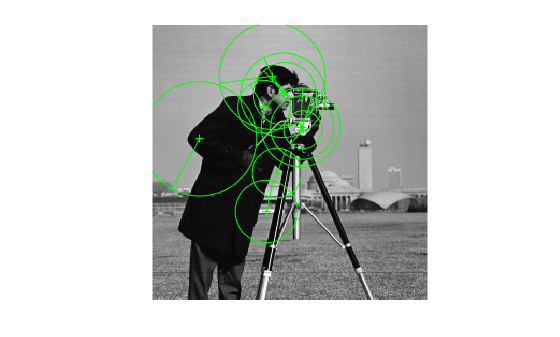# plot

## Syntax

``plot(points)``
``plot(points,ax)``
``plot(points,ax,Name=Value)``

## Description

example

````plot(points)` plots points in the current axis.```
````plot(points,ax)` plots points in the specified axis.```
````plot(points,ax,Name=Value)` specifies options using one or more name-value arguments in addition to any combination of arguments from previous syntaxes. For example, `plot(ShowOrientation=true)` renders the orientation in the display plot.```

## Examples

collapse all

Detect, extract, and plot KAZE points, including their orientation.

`I = imread('cameraman.tif');`

Detect KAZE points from the image.

`points = detectKAZEFeatures(I);`

Extract KAZE features from the detected points.

`[features,valid_points] = extractFeatures(I,points);`

Plot the 10 strongest points and show their orientations.

```imshow(I) hold on strongestPoints = selectStrongest(valid_points,10); plot(strongestPoints,'showOrientation',true) hold off```Extract SURF features from an image.

```I = imread('cameraman.tif'); points = detectSURFFeatures(I); [features, valid_points] = extractFeatures(I,points);```

Visualize 10 strongest SURF features, including their scales and orientation which were determined during the descriptor extraction process.

```imshow(I); hold on; strongestPoints = valid_points.selectStrongest(10); strongestPoints.plot('showOrientation',true);```` I = imread('cameraman.tif');`

Detect corner features.

` featurePoints = detectHarrisFeatures(I);`

Plot feature image with detected features.

``` imshow(I); hold on; plot(featurePoints);```## Input Arguments

collapse all

Points, specified as a points object. The object contains information about the feature points detected in the 2-D grayscale input image.

Handle to use for display. You can set the handle using `gca`.

### Name-Value Arguments

Specify optional pairs of arguments as `Name1=Value1,...,NameN=ValueN`, where `Name` is the argument name and `Value` is the corresponding value. Name-value arguments must appear after other arguments, but the order of the pairs does not matter.

Before R2021a, use commas to separate each name and value, and enclose `Name` in quotes.

Example: `plot(ShowOrientation=true)` renders the orientation in the display plot.

Display scaled circle, specified as `true` or `false`. When you set this value to `true`, the object draws a circle proportional to the scale of the detected feature, with the feature point located at its center. When you set this value to `false`, the object turns the display of the circle off.

The algorithm represents the scale of the feature with a circle of `6`*`Scale` radius. The algorithm uses this equivalent size of circular area to compute the orientation of the feature.

Display feature point orientation, specified as `true` or `false`. When you set this value to `true`, the object draws a line corresponding to the point's orientation. The object draws the line from the feature point location to the edge of the circle, indicating the scale.

## Version History

Introduced in R2011b